#### A PHP Error was encountered

Severity: Notice

Message: Trying to get property of non-object

Line Number: 17

Backtrace:

Line: 17
Function: _error_handler

File: /home/publishers12/public_html/application/controllers/Articleviews.php
Line: 60
Function: view

File: /home/publishers12/public_html/index.php
Line: 318
Function: require_once

#### A PHP Error was encountered

Severity: Notice

Message: Trying to get property of non-object

Line Number: 18

Backtrace:

Line: 18
Function: _error_handler

File: /home/publishers12/public_html/application/controllers/Articleviews.php
Line: 60
Function: view

File: /home/publishers12/public_html/index.php
Line: 318
Function: require_once

#### A PHP Error was encountered

Severity: Notice

Message: Trying to get property of non-object

Line Number: 19

Backtrace:

Line: 19
Function: _error_handler

File: /home/publishers12/public_html/application/controllers/Articleviews.php
Line: 60
Function: view

File: /home/publishers12/public_html/index.php
Line: 318
Function: require_once

#### A PHP Error was encountered

Severity: Notice

Message: Trying to get property of non-object

Line Number: 20

Backtrace:

Line: 20
Function: _error_handler

File: /home/publishers12/public_html/application/controllers/Articleviews.php
Line: 60
Function: view

File: /home/publishers12/public_html/index.php
Line: 318
Function: require_once

Stress wave interactions in cracked rocks for the investigation of oil extraction

#### A PHP Error was encountered

Severity: Notice

Message: Trying to get property of non-object

Line Number: 35

Backtrace:

Line: 35
Function: _error_handler

File: /home/publishers12/public_html/application/controllers/Articleviews.php
Line: 60
Function: view

File: /home/publishers12/public_html/index.php
Line: 318
Function: require_once

">

#### A PHP Error was encountered

Severity: Notice

Message: Trying to get property of non-object

Line Number: 36

Backtrace:

Line: 36
Function: _error_handler

File: /home/publishers12/public_html/application/controllers/Articleviews.php
Line: 60
Function: view

File: /home/publishers12/public_html/index.php
Line: 318
Function: require_once

">

## "Stress Wave Interactions in Cracked Rocks for the Investigation of Oil Ex-traction"

https://www.gavinpublishers.com/journals/journals_details/" target="_blank">  Home
• #### A PHP Error was encountered

Severity: Notice

Message: Trying to get property of non-object

Filename: views/article_view.php

Line Number: 39

Backtrace:

File: /home/publishers12/public_html/application/views/article_view.php
Line: 39
Function: _error_handler

File: /home/publishers12/public_html/application/controllers/Articleviews.php
Line: 62
Function: view

File: /home/publishers12/public_html/index.php
Line: 318
Function: require_once

• " target="_blank">   Board Members
• #### A PHP Error was encountered

Severity: Notice

Message: Trying to get property of non-object

Filename: views/article_view.php

Line Number: 40

Backtrace:

File: /home/publishers12/public_html/application/views/article_view.php
Line: 40
Function: _error_handler

File: /home/publishers12/public_html/application/controllers/Articleviews.php
Line: 62
Function: view

File: /home/publishers12/public_html/index.php
Line: 318
Function: require_once

• " target="_blank">   Article In Press
• #### A PHP Error was encountered

Severity: Notice

Message: Trying to get property of non-object

Filename: views/article_view.php

Line Number: 41

Backtrace:

File: /home/publishers12/public_html/application/views/article_view.php
Line: 41
Function: _error_handler

File: /home/publishers12/public_html/application/controllers/Articleviews.php
Line: 62
Function: view

File: /home/publishers12/public_html/index.php
Line: 318
Function: require_once

" target="_blank">   Current Issue
• #### A PHP Error was encountered

Severity: Notice

Message: Trying to get property of non-object

Filename: views/article_view.php

Line Number: 42

Backtrace:

File: /home/publishers12/public_html/application/views/article_view.php
Line: 42
Function: _error_handler

File: /home/publishers12/public_html/application/controllers/Articleviews.php
Line: 62
Function: view

File: /home/publishers12/public_html/index.php
Line: 318
Function: require_once

" target="_blank">   Archive
• #### A PHP Error was encountered

Severity: Notice

Message: Trying to get property of non-object

Filename: views/article_view.php

Line Number: 43

Backtrace:

File: /home/publishers12/public_html/application/views/article_view.php
Line: 43
Function: _error_handler

File: /home/publishers12/public_html/application/controllers/Articleviews.php
Line: 62
Function: view

File: /home/publishers12/public_html/index.php
Line: 318
Function: require_once

" target="_blank">  Article Process Fee
• #### A PHP Error was encountered

Severity: Notice

Message: Trying to get property of non-object

Filename: views/article_view.php

Line Number: 44

Backtrace:

File: /home/publishers12/public_html/application/views/article_view.php
Line: 44
Function: _error_handler

File: /home/publishers12/public_html/application/controllers/Articleviews.php
Line: 62
Function: view

File: /home/publishers12/public_html/index.php
Line: 318
Function: require_once

" target="_blank">   Special Issue
• Panayiotis A. Kakavas-Papaniaros1*, Maria P. Kakavas2

1Department of Civil Engineering, Technological Educational Institute of Western Greece, Greece

2Department of Geology, University of Patras, Greece

*Corresponding author: Panayiotis A. Kakavas-Papaniaros, Department of Civil Engineering, Technological Educational Institute of Western Greece, Greece. Email: kakavas@teiwest.gr

Received Date: 28 May, 2018; Accepted Date: 28 May, 2018; Published Date: 05 June, 2018

1.       Summary

Two-dimensional numerical techniques have been applied to obtain solutions of problems of stress wave interactions with cracks in rock media. Finite-difference Lagrangian programs that incorporate a comprehensive elastic-plastic-hydrodynamic behavioral model, have utilized as basic numerical programs. Significant capabilities of such codes are:

(a)     the treatment of antiplane shear, or out-of-plane displacement (where the motion is dependent only on the in-plane coordinates) and

(b)    the incorporation of a dynamic brittle fracture criterion (known as the Griffith criterion).

In-plane problems the propagation of a stress wave through a semi-infinite space containing

(a)     a semi-infinite crack,

(b)    and a finite stationary crack is considered.

The crack can be oriented at a 30o angle with the wave front in these problems. The computations demonstrated that it is possible to observe the diffraction of the shear wave fronts and to follow the flow of energy through the crack.

An ideal but relatively simple problem was chosen, in this study, as a test vehicle for the out-of-plane program, namely the antiplaque shear loading of a crack. In the case of a stationary crack, the numerical solution was shown to give excellent agreement in all respects with the analytical solution. Using the Griffith criterion, moderately good agreement was obtained for the case of the running crack. It is necessary to examine the dampen oscillations at the wave front by the incorporation of a fictive viscosity. In the case of pressure, a quadratic viscosity component is added. In the case of shear, a linear term is normally added. A linear viscosity tends to increasingly spread the wave front, however. A study of the origins of this viscosity term has led to the development of an alternate formulation for the viscosity term which is cubic and applies both to pressure and shear. This alternate formulation will eventually replace the so-called anisotropic or Navier Stokes linear viscosity.

2.       Keywords: Cracked Rocks; Finite Difference Method; Fracture Mechanics; Wave Propagation

1.       Introduction

For some cracked rocks, different methods of calculating velocities and the effects of pore fluids are preferable. Numerous theories have been developed to describe the effects of crack-like pores. Eshelby  examined the elastic deformation of elliptical inclusions, and these results were then applied to the compressibility of rocks. In concept, long, narrow cracks are compliant and can be very effective at reducing the rock moduli at low crack porosities. The primary controlling factor for elliptical fractures is the aspect ratio, α, defined as the ratio of the ellipse semi minor.

(a)     to semi major

(b)    axes.

The smaller the value of α, the softer the crack and cracked rock, resulting in lower velocities and stronger pressure dependence.

Numerous assumptions are made in the derivation and application of cracked media models, such as the following:

(1)    Porous material is isotropic, elastic, mono mineralic and homogeneous

(2)    Fracture population is dilute, and few, or only first-order, mechanical interactions occur among fractures

(3)    Fractures can be described by simple shapes

(4)    Pore-fluid system is closed, and there is no chemical interaction between fluids and rock frame (however, shear modulus need not remain constant).

Some of these assumptions may be dropped, depending on the model involved. For example, Hudson (1990)  specifically includes the effect of anisotropic crack distributions. One particularly useful result was derived . Using scattering theory, they derived the general relation of bulk and shear moduli of the cracked rock to the crack porosity, aspect ratio, mineral, and inclusion or crack moduli .

Rock at depth is subjected to stresses resulting from the weight of the overlying strata and from locked in stresses of tectonic origin. When an opening is excavated in this rock (such as a wellbore), the stress field is locally disrupted and a new set of stresses are induced in the rock surrounding the opening. The in-situ "Lithostatic" stresses are usually unequal. Such different stresses are required or faults, folds, and other structural features would never be developed. In contrast, most laboratory data are collected under equal stress or "hydrostatic" conditions. Differential or triaxial measurements are comparatively rare [5-8].

In a simple compacting basin with neither lateral deformation nor tectonic stresses, the vertical stress will be largest. Lateral stresses will be developed in a basin as sediments are buried and compacted but are constrained horizontally. Both uniform hydrostatic and unequal lithostatic stress conditions. Relying on Fracture Mechanics and Statistical Physics,  introduced a few key concepts, which allow understanding and quantifying how cracks do modify both the elastic and transport properties of rocks. The main different schemes, which can be used to derive the elastic effective moduli of a rock, were presented. It was shown from experimental results that an excellent approximation is the so-called non-interactive scheme. The main consequences of the existence of cracks on the elastic waves are the development of elastic anisotropy, due to the anisotropic distribution of crack orientations, and the dispersion effect, due to microscopic local fluid flow. Two macroscopic fluid flow regimes can be distinguished:

(i)                  the percolative regime close to the percolation threshold and

(ii)                the connected regime well above it.

Application of uniaxial stress to a sample of granite causes elastic wave velocity anisotropy . Compressional waves travel fastest in the direction of the applied stress. Two shear waves travel with generally different speeds in any direction, exhibiting acoustic double refraction which increases with increasing stress. Most transport and mechanical properties of rocks, such as electrical resistivity, thermal conductivity, hydraulic permeability, and compressibility and the velocity and attenuation of acoustic waves are strongly influenced by the presence of microcracks and fractures in the rock and by the fluids contained in these cracks .

Knowledge of the presence and properties of microcracks in rocks and their relationship to the mechanical and transport properties therefore has considerable practical importance. For example, the exploration for and the exploration of mineral, hydrocarbon and geothermal resources depend on the in situ measurement of many of the physical parameters previously mentioned. The underground isolation of hazardous wastes depends on the ability to select sites where the transport properties of the rocks are favorable.

Numerical methods can provide extremely powerful tools for analysis and design of engineering systems with complex factors that are not possible or very difficult with the use of the conventional methods. Various numerical methods have been employed to study elastic wave scattering problems, including Maslov theory , the finite difference method , the finite element method , the Born approximation , the complex screen approach , Kirchhoff-Helmholtz integration , pseudo-spectrum method  and the boundary element method .

A 2-D Boundary Element Method (BEM) program to model elastic wave excited by a point explosive source propagating in cracked rocks have used by Han & Cao 2014 . Effects of different crack parameters, such as crack scale length and crack density were analyzed. Using waves as a tool often means that rock properties are determined or the interior of a sample is studied without being damaged. Another use of waves is to measure the velocities in rock samples shaped as cubes, spheres or cores with plane and parallel end surfaces in order to determine if the rock is anisotropic; an important property to for example for the evaluation of stress measurements. Wave propagation in rock as a tool for determination of rock properties and as a consequence of activities, such as trains .

Direct laboratory observations as a framework for discussing the physics of intersonic shear rupture occurring in constitutively homogeneous (isotropic and anisotropic) as well as in inhomogeneous systems, all containing preferable crack paths or faults were provided by Rosakis (2002) . The damage mechanics formulation by incorporating theoretical and experimental dynamic crack growth laws that have been shown to be valid over a wide range of loading rates was recently extended.

2.       Mathematical Background

Elastic waves are comprised of compressional (or P-waves) and shear (or S-waves). In compressional waves, the particle motion is in the direction of propagation. In shear waves, the particle motion is perpendicular to the direction of propagation. Understanding the velocity of these waves provide valuable information about the rocks and fluids through which they propagate. Stress strain relationships in rocks considered only the static elastic deformation of materials. By adding the dynamic behavior, one arrives at how elastic waves propagate through materials. If a body is changing its speed as well as deforming, there will be an unbalanced force because of the acceleration described through Newton’s second law: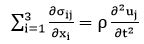(1)

where ρ is the density,  is the displacement, and t is the time. Combining this with Hook’s Law gives the general wave equation. For a plane wave in the  direction, this can be written as: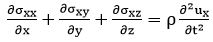(2)

However, if the material is being deformed, we will have strains associated with the change of displacement with position. In turn, these strains can be related to the stresses through the appropriate modulus, M: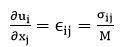(3)

For constant elastic components, this simplifies to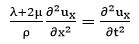(4)

The solution to this equation gives the compressional velocity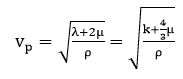(5)

Similarly, for shear motion: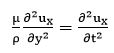(6)

and we get the shear velocity: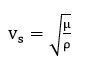(7)

In 3-D inhomogeneous isotropic elastic media, the propagation of elastic waves is governed by the momentum equation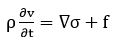(8)

and the generalized stress-strain relationship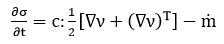(9)

where v=(vx, vy, vz) is the particle velocity vector, σ is the stress tensor, the components of which are σij, i, j (x, y, z), ρ is the mass density, c is the fourth-order elastic tensor,  is the moment density, over which the dot means the time derivative and the superscript T represents the transpose operator.

3.       Problem Area

Excavation processes in rock media typically involve the action of strong dynamic stresses introduced either from explosive, mechanical, or other impulsive loading sources. The propagation of stress waves in homogeneous, isotropic media is reasonably well understood. In-situ rock media, however, typically contain large scale discontinuities in the form of cracks, joints, and faults. Interactions of stress waves with these discontinuities can cause slippage along the cracks, or extension (propagation) of the cracks, or separation. The interactions can also alter the characteristics of the stress wave transmitted across the discontinuity.

This paper is concerned with an analysis of the detailed mechanisms involved when strong stress waves interact with the crack surfaces in jointed rock media. Of particular interest are cracks which are obliquely oriented relative to the wave front. The understanding thus obtained can contribute to the advancement of knowledge of excavation processes in various media, and how to control and/or improve such processes. The technical approach used to study the details of stress wave- I crack interactions was based on two-dimensional numerical analyses of the dynamic phenomena occurring under various conditions of stress wave loading and crack orientation relative to the wave front. A major difficulty in examining wave interactions with discontinuities such as cracks or fracture surfaces arises due to the constraint of the continuum model which is normally assumed in numerical analyses of wave propagation. This work is divided into the following two tasks, corresponding to analyses of stress wave interactions with,

(a)     Single, semi-infinite cracks and

(b)    Single, finite-length cracks.

3.1    Interaction of Stress Waves with Single, Semi-Infinite Cracks

This task was concerned with the analysis pertaining to cracks which are semi-infinite in extent, of which intersect with the ground surface. The work initially consisted of the selection and determination of the problem specifications (such as media properties, loading wave characteristics, and crack orientation and condition). Finite difference code solutions of the problems defined were then set up and run. These solutions provide complete quantitative data for all the state and motion variables of interest throughout the computing field at regular intervals of time. In addition, spatial plots of the principal stress, particle velocity, and displacement fields were numerically obtained at selected times during the event. Following completion of the solutions, analysis and interpretation of the results were performed to characterize the transmitted stress waves.

3.2    Interaction of Stress Waves with Single, Finite Cracks

This task was concerned with cracks of finite length, so that the interaction of a stress wave with a crack tip could be examined. The problems were selected so that comparisons between the cases of semi-infinite and finite cracks could be made. Also included in this task was the development of a dynamic Griffith fracture criterion, to enable the study of crack propagation. In conjunction with this, the finite difference code was generalized to accommodate antiplane shear, or out-of-plane displacement. To verify the suitability and accuracy of the code, and modifications made there during the course of the program, for analyses of wave/ crack interactions, analytic solutions of selected test problems were obtained and used to check out corresponding numerical solutions obtained with the code.

3.3    Major Accomplishments

Primary program accomplishments included

a)       The completion of finite difference code solutions of three in-plane stress wave/crack interaction problems.

b)       The codification of the equations of anti-plane shear (z-independent) to provide a new capability for finite difference code analyses.

c)       The formulation of a dynamic Griffith criterion for analyses of crack propagation with the code.

These Items are summarized in the following sub-sections.

3.3.1           In-Plane Numerical Solutions

The rock medium selected for these problems was granite. The material properties assumed for the granite are described in Table I.

The subscript ‘0’ in the above indicates that these are normal, pre-shocked values. Three problems of wave interactions with cracks were selected for analysis by means of code solution, as depicted in Figure 1. These problems were chosen to demonstrate the utility of numerical techniques for obtaining detailed information on the response of cracked media subjected to impulsive loads, in general, and, in particular, for assessments of the wave interactions in the vicinity of a crack. It is noted that the explicit definition of a crack surface such as specified in these problems is not generally amenable to treatment through conventional code techniques, which normally assume a continuous material model. The problems were run in plane geometry, assuming plane strain; the variables are thus independent of the z-coordinate (perpendicular to the cross-section shown). The first problem selected for analysis (Case I)-consisted of interactions of a stress wave with a crack oriented at a 30° angle with the wave front.

The stress wave was generated by uniformly loading the left face of the granite block with a pressure pulse. A triangular pressure pulse of 500 Pa peak magnitudes and 0.2 m sec duration was used for this problem, as sketched below (Figure 2)

The crack in this problem was characterized by a free slip condition and zero width. Opening of the crack was allowed to occur if stress components normal to the crack went into tension. The second problem considered (Case II) involved the interaction of a stress wave with a finite-length crack. The angle of orientation of the crack and the applied pressure loading were the same as in the first problem. The crack extended from the loading surface (lower left) to the crack tip, situated on the horizontal mid-plane of the block. The finite difference code solutions of these problems were successfully completed. Plots depicting the particle velocity field and the principal stress field occurring in the test block were obtained for several times during the interactions.

3.3.2           Dynamic Griffith Criterion

In connection with efforts made under this paper to enable the study of propagation of cracks under stress wave loading, the incorporation of equations into the finite difference code which govern the rate of propagation of a brittle crack surface in an elastic material was undertaken. These equations are known as the Griffith criterion [24-26] and they provide a relation between power input to the body and the rate of uptake of this power by strain energy, kinetic energy, and new surface energy . The concept of surface energy is the feature that was introduced by Griffith and it requires the determination of an additional material parameter, namely the surface energy per unit area. An algorithm appropriate for the finite difference code was programmed and tested on a model problem. The results indicated that the effective crack propagation was slower than predicted theoretically. It is expected that improved results would be obtained with an alternate form of the active viscosity.

3.3.3           Analytical Comparison Problems

To verify the finite difference code solutions and the formulation changes made, comparisons of numerical results with analytical solutions of model problems were made. As part of this effort, the capability was added to the code for the treatment of anti-plane shear, or out-of-plane displacements, with the restriction that the motions are independent of the z-coordinate, so as to retain the two-dimensional character of the code. This was done primarily since the only elasto-dynamic solutions currently available for an accelerating crack are those for the case of anti-plane shear, although it also represents a useful tool in numerical analysis which has been unavailable.

A model problem of simple, shear motion of a slab was first solved with the modified code. The results of the finite difference code solution showed excellent agreement with the analytical solution for this case. A full, two dimensional problem involving the interaction of an anti-plane shear wave with a stationary crack was then set up and run. Excellent agreement with the analytical solution was also achieved for this case.

4.       Numerical Solutions of In-Plane Problems

4.1    Finite Difference Lagrangian Method

The effectiveness of a finite difference scheme for solving problems in continuum mechanics is demonstrated by a series of problems ranging from elasticity theory to gas dynamics [28,29]. Von Neumann and Richtmyer , proposed the Artificial Viscosity Method (AVM) for calculating problems in hydrodynamics. The technique was described for one-dimensional flow with the Lagrange form of the hydrodynamic differential equations. The region of flow was divided into a finite mesh of grid points at which the various parameters could be specified. The differential equations were then approximated by a second-order-difference scheme involving these grid-point parameters. It was found that the quadratic artificial viscosity used to treat shocks did not provide sufficient damping for non-physical oscillations that usually occurred in the grid. However, this difficulty is easily overcome by incorporating a linear viscosity to stabilize the grid. The result is a second-order-difference scheme that gives very accurate results for a large range of problems in one space dimension. The artificial viscosity is included in Euler and Lagrange codes for two reasons:

(a)     allow a code with a continuum formulation to handle shock waves, which, mathematically, are discontinuities

(b)    provide grid stabilization for quadrilateral and hexahedral elements which use one point reduced evaluation of the element constitutive model.

When the finite mesh and artificial viscosity concept is extended into two space dimensions, many different ways of formulating the differential equations and the finite difference equations result. Also, the additional degree of freedom I n the mesh permits an increased possibility for nonphysical grid distortion, analogous to the non-physical oscillations in the one-dimensional problem. The additional degree of freedom in the mesh permits an increased possibility for nonphysical grid distortion, analogous to the non-physical oscillations in the one-dimensional problem. The problem of grid distortion plus the possibility of formulating the equations in many different ways has led to a search for the best method of solving problems in hydrodynamics using the original von Neumann-Richtmyer idea. One of the approaches taken is to damp spurious signals in the grid by including more grid points in the difference operators.

The finite difference operators that replace the partial derivatives are formulated so that they have an important bearing on the physics that the set of partial differential equations is meant to describe. Space derivatives are defined as the summation of the normal component of a flux around an enclosed area thus, conservation form is introduced into the difference equations. The two-time step and the two-grid system of von Neumann-Richtmyer are employed. One grid system is used to calculate gradients of pressure stresses and the other to calculate the divergence of the velocity vector. The pressure gradients are centered at grid nodes at integral times intervals. The divergence of the velocity is centered at mid-points on the grid at g-time intervals (Figure 3)

In 2-D Cartesian coordinates the terms in the difference equations that represent the partial derivatives in the left side of Eq. (10) below will collect and be identically equal to the time centered difference of the quantities on the right side of each equation.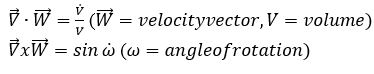(10)

As noted above, numerical solutions of three problems involving the interaction of stress waves with cracks were performed [28,29].

4.2    Physical Model

The computer program used in this study was a two-dimensional finite difference code, which solves the equations of motions for elastic-plastic bodies by means of a finite-difference Lagrangian-cell technique. The mathematical formulation is basically the same as that described by Wilkins [28,29]. To delineate the boundary between elastic and plastic deformations, various yield criteria may be used, such as von Mises, Mohr-Coulomb, or arbitrary functions. Within the chosen yield surface, the deformations are considered to be elastic, i.e., when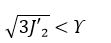(11)

where J2 is the second invariant of the deviatoric stress tensor and  is the yield strength. Excursions on the yield surface can be made in accordance with either the Prandtl-Reuss or plastic potential flow rules. To model a crack surface, the finite difference code contains provisions for inserting surfaces of discontinuity, which consist of grid lines having a dual set of lattice points. These surfaces are discussed in the following section.

4.3    Surfaces of Discontinuity

In a normal Lagrangian computational grid, material elements on either side of an interface at any point are coupled to each other for the entire problem; they are, i.e., locked or welded together along the line segment connecting any two lattice points along the interface. At any interface, which may represent a crack within a material or the boundary between two different materials, there are, however, in general, special boundary conditions which apply, and, in addition, there is the possibility of forces which may be set up that tend to cause the materials to slip past each other or to separate. A gas flowing past a metal surface is an example of such a case. The onset of material fracture during a problem also gives rise to the requirement for treating the decoupling or uncoupling of elements which are, in this case, within an originally competent material. For application to problems in fracture mechanics the latter requirement is particularly important.

The numerical algorithms that are used in hydro codes to solve the governing partial differential equations are derived using either finite element or finite difference methods. Under certain circumstances the two methods give identical algorithms. The Lagrangian form of the conservation equations leads to Lagrangian codes in which the mesh is determined by the material and deforms with it, whereas the Eulerian mesh is fixed. The Lagrangian codes are much more efficient to run, the mesh becomes distorted after a critical plastic strain and their predictions lose their accuracy. The Eulerian codes can handle large deformations very well but present unique problems. Here we follow the Lagrangian technique.

A formulation of sliding interfaces for Lagrangian codes, as reported by Wilkins [28,29], provided a capability for the numerical treatment of problems involving sliding of two materials along an interface. This formulation served as the basis for development of the surface of discontinuity capability currently available in the code. The basic features of the surface of discontinuity formulation are illustrated in Figure 4. The grid line corresponding to the surface of discontinuity is known in common balance as a slide line. At the start be the lattice points along the slide line may be individually designated as decoupled points, corresponding to their lying on an interface, or as coupled points, in which case their behavior is the same as in an ordinary mesh. For decoupled points, special sets of governing equations are used to individually determine the motion of the point pairs, to reflect the fact that there is an interface, such that, e.g., shear stress cannot be supported. If forces are present which tend to cause slippage, the decoupled points will thus disengage and move separately along the slide line.

Additionally, the development of tensile stresses normal to an interface will tend to cause material separation and formation of voids. Provisions have been made in the code to treat this phenomenon, also. The void opening test is made by computing the stress normal to the interface at a decoupled slide point and comparing this value with a selected critical value of stress required for uncoupling. If the computed stress is greater than the critical value, in tension, then that point is designated as a free surface point. The newly formed free point is then moved in accordance with the regular equations of motion for a point on a free surface.

For the problems performed in this study, the critical value for uncoupling was set to zero, such that any tensile stress would tend to cause separation. In other applications, e.g., for the interfaces in laminated materials, this value could be equal to the bond strength. Void closure may also occur and is treated in the code by appropriate tests to determine if the materials have come in contact. If so, the equations of the surface of discontinuity are restored, and the materials may subsequently slip or re-open, as before. Lattice points along the slide line which are initially designated as coupled points may dynamically decouple, individually, during the course of a problem, if a selected criterion is met. Various decoupling, or fracture, criteria may be used. Once the lattice points are decoupled, the equations of the surface of discontinuity are invoked. A mechanism is thus provided which can be used to model crack propagation within a material.

The equation of state of granite, suitable for the low-pressure regime applicable in these problems, was formulated as follows: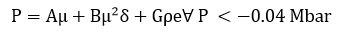(13)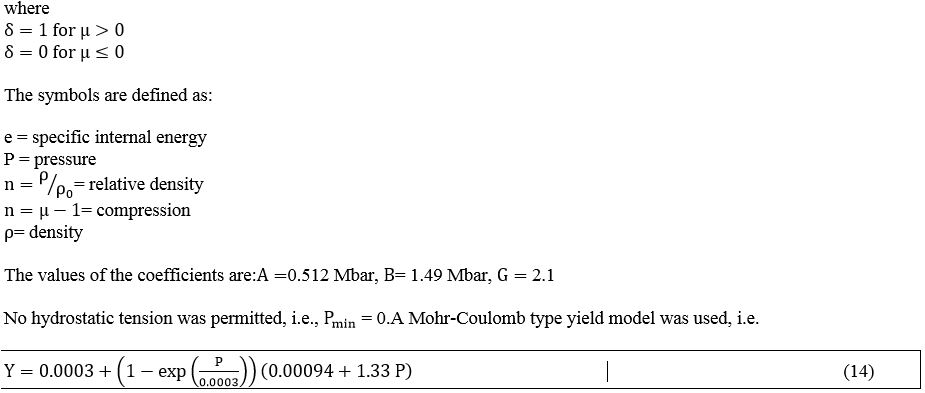where  is the yield strength, in Mbars. The maximum value permitted for  was 10 kbars.

Stress  - time profiles at points A, D, E, and F are shown in Figure 9. The peak stress in the transmitted wave (points D, E; and F) is seen to be reduced by about 25% from that in the incident wave. The aforementioned two-wave structure in the transmitted wave and the reflected wave at point A are also displayed in these plots

The spatial trajectory of the point pair (W, X) on the crack surface during the time span of the solution is plotted in Figure13.

5.       Conclusions and Discussion

The present paper deals with the stress wave interaction in cracked rocks. In case I, the interaction of stress wave with single, semi-infinite crack was considered. The finite difference numerical approach has shown that the material slippage along the crack at x=100 cm and y=0, increases almost linearly from 0.18 msec up to 0.50 msec and after this time remains constant up to 0.9 msec. The relative displacement reaches the maximum value of 0.16 cm at t=0.5 msec. At the left point of the crack (point B) the displacement drops while to the right (point C) the displacement reduces more rapidly. At time 0.50 msec the displacement left and right points (B and C) increases. The difference in the displacement at this time is 0.16 cm. The displacement at the points A,D,E,F that are further than the points near the crack (B,C) shows similar behavior but the points D,E that are near the crack shows higher displacement than the point F that is far from the crack.

The time history of the stress profiles at four points along y=0 cm, shows a decay vibration with a time delay 0.20 msec from point A to point D. The delay reduces to 0.10 msec as the stress wave propagates from point D to E and E to F. The σxx value of stress is negative as produces compressive waves along its path.

In the second case (case II) the interaction of stress wave with single finite length crack with crack growth was examined. This study have shown that the relative displacement at the points near the crack increases rapidly, almost linearly, at the points (W, X). At the points (R, S) and (M, K) the displacement shows a redaction at time 0.60 msec and after this time increases. At the first pair of points (W, X) the maximum relative displacement is 0.25 cm, while at the other pairs (R,S) and (M,N) is 0.16 and 0.13 msec respectively. Left and right along the crack the relative displacement is negative and the values are larger to the right than those to the left of the crack.

In case II, the spatial trajectories at the opposite points W, X show that to the left of the crack, point W, the trajectory increases negatively as the time increases up to 0.25 msec and next decreases. At point X, the trajectory increases up to t=0.24 msec and next continue to increases but returns back to x-axis. At the points D, H, L the y-displacement increases negatively with larger values at the point near the crack. At the points A, E, O, T where x=200 cm, the y-displacement increases with maximum values at point T that is located near the crack. Similar behavior occurs at the points B, F,J,P,U where in this case up to time t=0.40 msec no y-displacement takes place. Similar behavior occurs at the vertical points along the line x=300 cm. The σxx time profile at x=200 cm shows a Gaussian type distribution along the points A, I,E,O,T. Similar behavior one observes along the points C,G,V,Q,K.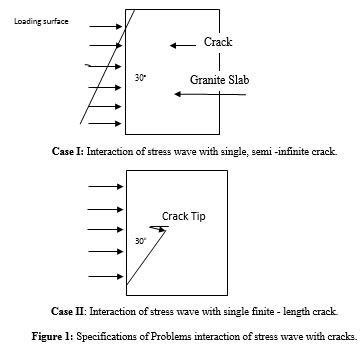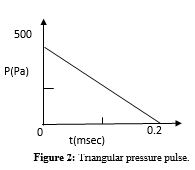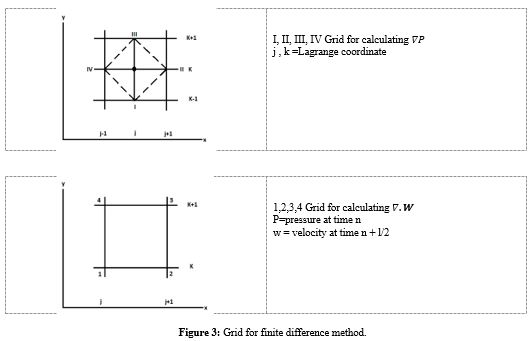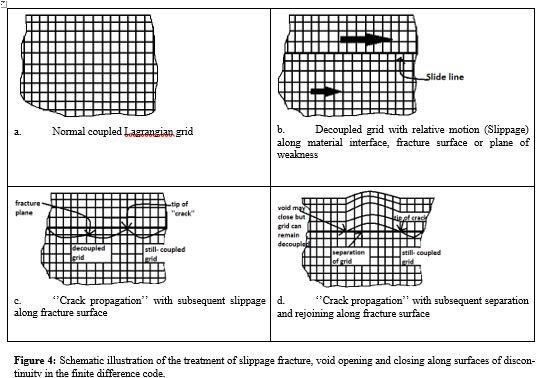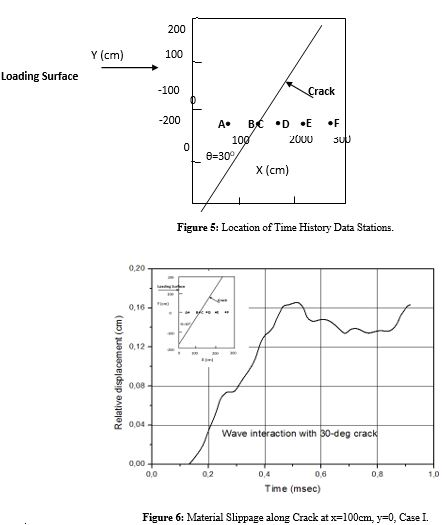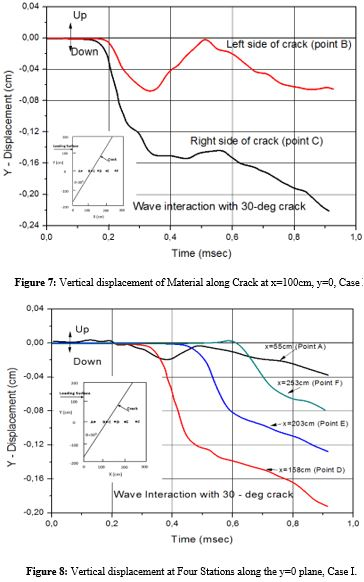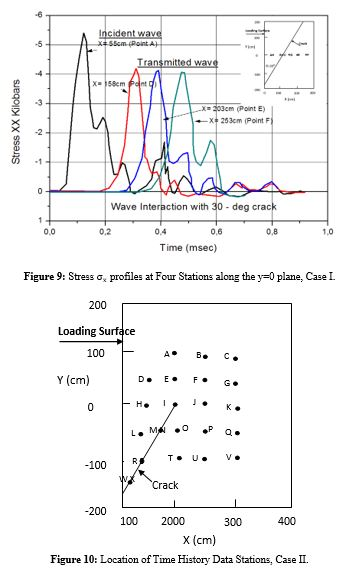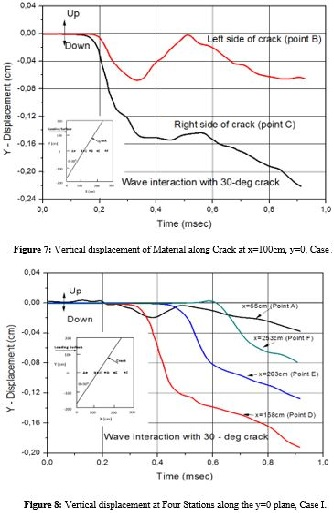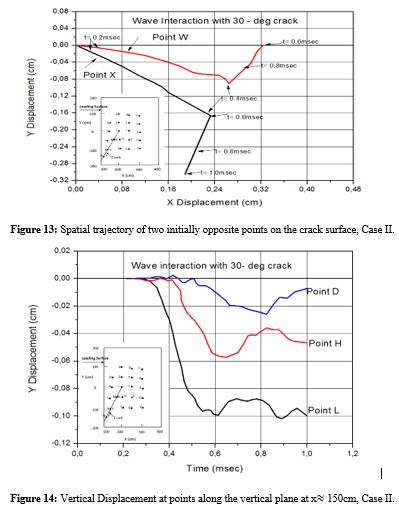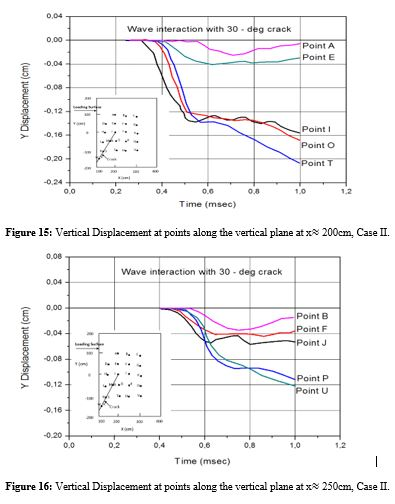Density ρ0=2690 Kg/m3 Dilatational Velocity Vd0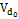= 5790m/sec Shear Velocity Vs0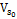= 3300 m/sec Bulk Modulus K0 = 0.512 (105) Pa Shear Modulus G0 = 0.293 (105)Pa Poisson's Ratio ν0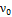= 0.26

Table 1: Material properties of rock medium (granite).

Citation: Kakavas-Papaniaros PA, Kakavas MP (2018) Stress Wave Interactions in Cracked Rocks for the Investigation of Oil Extraction. Arch Oil Gas Res: AOGR-103. DOI: 10.29011/ AOGR-103. 000003